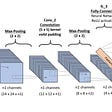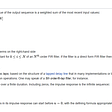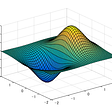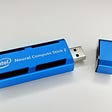# Adding Expand and Repeat Ops into OneFlow

Written by Liang Depeng; Translated by Wang Kaiyan, Dong Wenwen

This article introduces how to add the expand and repeat ops in the OneFlow, and you can have an insight into the features of the OneFlow after reading.

# ONEFLOW. TENSOR. EXPAND

`oneflow.expand(input, *sizes)`

The function of the `expand` is to copy the input tensor along dimensions of size 1, with the number of copies determined by the second parameter (which will be called `expand_size` below).

Here are some conventions for setting `expand_size` :

• The dimension of the `expand_size` is greater than or equal to the input tensor, and if it is greater than the input dimension, the dimension of output will increase.
• For dimensions whose input tensor is `1`, the dimension of `expand_size` can be set to be greater than or equal to `1`.
• For dimensions whose input tensor is not `1`, the dimension of `expand_size` can only be set to be equal to that of the input or `-1`.
• The newly added dimension can only be appended at the front and cannot be set to `-1`. Adding a new dimension is equivalent to copying the entire input tensor.

Example 1：

Example 2：

# Implementation in a Single Card

The next section describes the idea of implementing the `expand` from a single card perspective, i.e., the distributed case is not considered.

From the introduction of the previous section, we know that the value at a certain position of the output tensor of the `expand` is copied from the corresponding position of the input tensor, so the key point lies in how to map the index at a certain position of the output to the index at the corresponding position of the input.

Before introducing how to compute the index mapping, let’s first review the concept of the `stride `property of a tensor. For an `n`-dimensional tensor that is continuous in memory, the `stride` property can be used to quickly locate the one-dimensional index corresponding to the index `(x, y, z, k)` at any position of the tensor.

Example:

The value of `stride` for each dimension represents the step length that should be moved in the memory for every one increase in the index of that dimension. The formula for calculating every dimension of `stride` is as follows:

Example:

Next, let’s see how to compute the mapping of the output index of the `expand` to the input index.

We know that if a dimension of the input tensor is 1 and the dimension corresponding to `expand_size` is greater than 1, it is equivalent to copying the input tensor along that dimension. That is, for the copied dimension, whatever the index of the output dimension is, it corresponds to the index 0 of that dimension of the input tensor. In fact, a new `output_stride` is constructed by modifying the `stride` parameter of the input tensor, and the `output_stride` is calculated as follows:

• If the value of `i` in a dimension of `expand_size` is `-1` or the same as the corresponding dimension of the input tensor, then `output_stride[i] = stride[i]`
• If the value of `i` in a dimension of `expand_size` is greater than `1` and the corresponding dimension of the input tensor is 1, then `output_stride[i] = 0`
• For the case where the `expand_size` dimension is greater than the dimension of the input tensor, then `output_stride[i] = 0` for the newly added dimension `i`

Example of calculating `output_stride`:

For example:

# Implementation in Multi-Card via Consistent View

The next section describes how adding ops in OneFlow is different from that of other frameworks. In addition to correctly implementing the logic of computation in the single card perspective, it is also necessary to consider the logic of multi-card in consistent view, including the logic of output shape inference, the setting of the `sbp` signature and the logic of the actual computation.

I’ll start with a brief introduction to the concept of the consistent view:

`OneFlow proposes the concept of consistent view to simplify distributed training. In simple terms, in OneFlow's consistent view, a cluster is abstracted as a "supercomputing device". In this way, users do not need to care about the details of computation and communication in the cluster, but only about the logical data and computation. Users can conduct distributed training just like programming in a single machine with a single card.`

The concept of sbp:

`Sbp is a concept put forward by OneFlow that describes the mapping relationship between data in the consistent view and data on real physical devices in the cluster. It is a combination of the initials split, broadcast, and partial.`

• `Split`

It represents the tensor on the real physical device, which is obtained by splitting the tensor in the consistent view. The dimension needs to be specified during splitting, while tensors on real physical devices can be restored to obtain tensors of consistent view after connecting.

• `Broadcast`

It represents that the tensor in the consistent view has a complete copy on all real physical devices.

• `Partial`

It means that the tensor in the consistent view has the same shape as the tensor on the physical device, but for the value on the physical device, it is only a part of the tensor in the consistent view. Take partial_sum as an example, if we add up the tensor of all devices in the cluster by position, only then can we restore the tensor in the consistent view. In addition to sum, operations such as min, max, etc. are also applicable to partial.

More details can be found at: https://docs.oneflow.org/v0.5.0/parallelism/02_sbp.html

To add ops in OneFlow, the developer also needs to set which combinations of `sbp` signatures are supported for the op's inputs and outputs, which requires additional learning.

However, in the consistent view, the implementation logic of the op sometimes needs to be considered, where its computation on the real physical device is inconsistent with the logical computation (i.e., the consistent view).

For example, for the `expand`, it may be necessary to modify the logical `expand_size` passed in by the user when it is computed on a real physical device. The main reason is that the sbp signature of the `expand` supports `split` on the input.

Example:

Suppose the user sets the sbp of the input tensor to `split(3)` , that is, to split the last dimension, and sets the logical tensor to be placed on two cards, then the real physical shape on each card is:

Then for both `expand_size` and `output_stride` on the real physical device, the following changes need to be made:

Why does `expand_size` need to be changed?

In the consistent view, when the actual computation is performed on each physical device, the input size actually gets the physical shape after spliting.

However, for the above example, the physical shape of the input on each device becomes `[4, 3, 1, 1]`, and if `expand_size` still keeps the logical size `[2, 4, 3, 4, 2]` set by the user at this time, the output size on each device is `[2, 4, 3, 4, 2]`, and the corresponding logical shape of the output is `[2, 4, 3, 4, 4]`, then the last dimension of the output is more than the original one.

And since how the user sets up sbp is only known at runtime, both `expand_size` and `output_stride` need to be recalculated based on the actual input size before the calculation is done on the physical device.

# Usage Introduction

`oneflow.repeat(input, *sizes)`

The function of the `repeat` is the ability to replicate any dimension of the input tensor, with the number of copies determined by the second parameter (called `repeat_size` below).

Some conventions for setting `repeat_size` are described below:

• The dimension size of `repeat_size` is greater than or equal to the input tensor, if it is greater than the input dimension, it is equivalent to adding dimensions to the output
• The value of any dimension of `repeat_size` should be set to greater than or equal to `1`. Suppose a dimension is set to `n`, which is equivalent to copying `n-1` of the corresponding dimension of the input tensor.
• The newly added dimension can only be added at the beginning and cannot be set to a value less than 1. Adding a new dimension is equivalent to copying the entire input tensor. Suppose the new dimension is set to `n`, then it is equivalent to copying `n-1` copies of the input tensor
• The value of any dimension of `repeat_size` can also be set to `0`, but this case is not considered here

The size of each dimension of the output tensor is calculated as follows:

# Relationship with the `expand`

In fact, the `repeat` and the `expand` are actually related, that is, the `repeat` can be implemented by the `expand` .

For example:

Example 1:

Example 2:

Example 3:

From the above example, we know that the `repeat` operation can be replaced by `reshape` + `expand` + `reshape`, and the problem becomes how to calculate the input `reshape` size, `expand_size` and output `reshape` size based on `input_shape` and `repeat_size`.

Example:

This is a tricky implementation of the `repeat`. Since it is replaced by `reshape` and `expand`, there is no need to consider the sbp problem, but we still need to write a separate op for performance.

# References

Related articles：

Also, welcome to join our Discord group to discuss and ask OneFlow related questions, and connect with OneFlow contributors and users all around the world.

--

--

--

## More from OneFlow

OneFlow is a performance-centered and open-source deep learning framework.

Love podcasts or audiobooks? Learn on the go with our new app.

## TRAFFIC SIGN DETECTION AND CLASSIFICATION## K-Nearest Neighbors put across## What is FIR?## Model-Based Meta Reinforcement Learning## Building A SPAM Detector with Naïve Bayes and AdaBoost Machine Learning Classifiers in JupyterLab.## ML Governance — Prerequisites## An effective strategy for Hyperparameter Optimization using Grid-Search## Audio Classification using Wavelet Transform and Deep Learning## OneFlow

OneFlow is a performance-centered and open-source deep learning framework.

## The Development of Credit-based Flow Control (Part 2)## Strong Homomorphic Encryption## Sky Computing: Accelerating Geo-distributed Computing in Federated Learning## How to run OpenVINO™ with Neural Compute Stick 2 on Linux